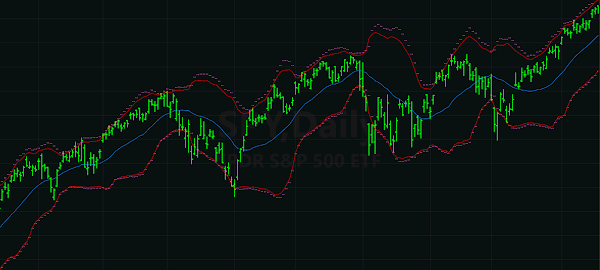### Bollinger Band prediction

The Bollinger Bands plot on a price bar chart is a common component of technical analysis among financial traders. Bollinger Bands display a graphical envelope around the moving average of price, with the width of the envelope representing volatility of the financial instrument charted.

Traders sometimes use Bollinger Bands for trading signals; for example, if a stock price crosses outside of the 2-standard-deviation envelope, buy or sell the stock. This is fine for signaling market orders, but if you want to set an intra-day stop order or a limit order at a specific price based on a Bollinger Band, you don't know where that price will be, because the band changes size during a single price bar as price moves in the range of the bar. Not only does the moving price affect the value of the moving average, but it also affects the standard deviation measurement.

First let's define some basic terms. The two components of a Bollinger Band are moving average $$\mu$$ and population standard deviation $$\sigma$$, which is the square root of the variance $$\sigma^2$$: $$\mu = \frac{1}{n} \sum_{i=1}^n x_i$$ $$\sigma^2 = \frac{1}{n} \sum_{i=1}^n (x_i-\mu)^2$$ or equivalently (making things simpler): $$\sigma^2 = \frac{1}{n} \left(\sum_{i=1}^n x_i^2\right)-\mu^2$$ where $$[x_1 ... x_n]$$ are the most recent $$n$$ closing prices.

The Bollinger Band envelope boundaries ($$B$$) are $$d$$ standard deviations ($$\sigma$$) from the moving average ($$\mu$$): $$B = \mu \pm d \sigma$$ where $$\mu$$ and $$\sigma$$ are dependent on the last $$n$$ values of $$x$$.

Because $$\mu$$ and $$\sigma$$ depend on the data values (prices), and the most recent price is constantly changing for the duration of the price bar, it's hard to figure out where the Bollinger Band and price would intersect.

So where will price intersect this moving target? We need to solve for $$x_n=B$$, where $$x_n$$ is the unknown future closing price of the bar.

Let's rewrite the mean and variance as:

$$\mu = \frac{s_1+x_n}{n}, \quad \sigma^2 = \frac{s_2+x_n^2}{n} - \mu^2$$ where $$x_n$$ is the unknown closing price, and $$s_1$$ and $$s_2$$ are the sums of the known $$n-1$$ values of $$x_i$$ and $$x_i^2$$, respectively:

$$s_1 = \sum_{i=1}^{n-1} x_i, \quad s_2 = \sum_{i=1}^{n-1} x_i^2$$

The cool thing is that while the closing price is varying up and down in the current bar, $$s_1$$ and $$s_2$$ are constants! The prices that made them have already occurred, they're in the past, and they don't change. That means a time-series algorithm doesn't need loops to calculate them (except for the first instance of each). For each new price bar, just subtract the oldest $$x_i$$ and add the newest. That makes the algorithm extremely efficient.

The future price that intersects the Bollinger Band is:

$$x_n = \mu \pm d \sigma$$

Expanding that with our rewritten mean and variance, that becomes:

$$x_n = \frac{s_1+x_n}{n} \pm d \sqrt{\frac{s_2+x_n^2}{n}- \frac{(s_1+x_n)^2}{n^2}}$$

I won't break down the steps in solving this for $$x_n$$. It suffices to say that you must move everything but the square root to the left side of the equation, square both sides to get rid of the square root, and then gather up the terms to solve for $$x_n$$ using the quadratic formula. It gets messy while working through it but the end result is rather simple and neat:

$$x_n = p_1 \pm d \sqrt{\frac{n \left(p_2 - p_1^2\right)}{n-1-d^2}}$$ where $$p_1 = \frac{s_1}{n-1}$$ $$p_2 = \frac{s_2}{n-1}$$

That's the closed-form solution for the future value of a price that would intersect a Bollinger Band.

The denominator $$n-1-d^2$$ looks troublesome, though. It could be zero. Or it could even be negative. At first I thought this was an artifact of my solution, so perhaps this expression was merely an indeterminate form that could be solved using L'Hôpital's rule, but after testing it with actual numbers, I realized that this is actually a limit beyond which there is no real solution.

For example, if $$n=10$$ and $$d=3$$, the denominator is zero. If you use a spreadsheet to generate 10 random numbers and calculate the Bollinger Band value 3 standard deviations out from the mean (using STDEVP in the spreadsheet for population standard deviation, not STDEV), and then change one of the numbers to be a huge outlier, the new Bollinger Band value gets even bigger. It is impossible for any outlier to go past it, although when the denominator is zero, an outlier can approach it closely if it's large enough. The situation is worse if the denominator is negative, say, $$n=9$$ and $$d=3$$; in that case, 3 standard deviations from the mean is always further beyond the biggest outlier in the population of $$n$$ values.

If our Bollinger Band width is 2 standard deviations from the mean, most of the prices would be inside the envelope, and we would expect the prediction where the price must go to intersect the Bollinger Band to be outside of the envelope. Likewise, when price does venture outside the 2-standard-deviation envelope, we would expect the prediction of where price must go to intersect the Bollinger Band to be inside the envelope. And indeed, that is what happens. Here is the same chart of SPY shown in the beginning of this article, with the intersection predictions plotted as tick marks, and they are outside the envelope except when the price ventures out of the envelope.Exchange-traded fund SPY showing 20-day moving average (cyan centerline) with 2-standard-deviation Bollinger Bands (red), and prediction of price-band intersection (magenta ticks). Charted by TradeStation 10.

Rounded to the nearest tick, this prediction can be used to place stop and limit orders.